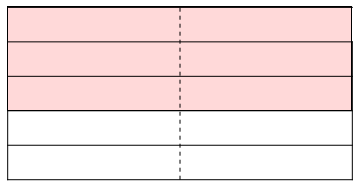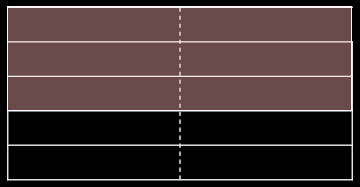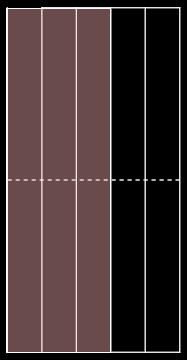# Multiplication of rational numbers (Math 0)

We’ve seen how to add and subtract pairs of rational numbers. But the natural numbers have another operation on them: multiplication.

Remember, a given rational number represents what we get when we cut an apple into pieces all of the same size, then take some number notePossibly more than we actually made, and possibly negative! of the little pieces. The product of $$\frac{a}{m}$$ and $$\frac{b}{n}$$ noteRecall that $$\frac{a}{m}$$ is “$a$ copies of the little-piece we get when we cut an apple into $$m$$ equal pieces. is what we call “$\frac{a}{m}$ multiplied by $$\frac{b}{n}$$”, and it answers the question “What happens if we do the procedure that would make $$\frac{b}{n}$$, but instead of starting by cutting one apple into $$n$$ pieces, we started by cutting $$\frac{a}{m}$$ apples into $$n$$ pieces?”.

We write the product of $$\frac{a}{m}$$ and $$\frac{b}{n}$$ as $$\frac{a}{m} \times \frac{b}{n}$$.

# Example

It’s hopefully easy to see that $$1 \times \frac{b}{n} = \frac{b}{n}$$. Indeed, the definition is “what do we get if we would make $$\frac{b}{n}$$, but instead of starting by cutting one apple, we started by cutting $$1$$ apple?”; but that’s just the same! It’s like saying “What if, instead of putting bread around my sandwich filling, I tried putting bread?”—I haven’t actually changed anything, and I’ll still get the same old sandwich noteor $$\frac{b}{n}$$ out at the end.

How about $$2 \times \frac{3}{5}$$? (Strictly speaking, I should probably be writing $$\frac{2}{1}$$ instead of $$2$$, but this way saves a bit of writing.$$\frac{2}{1}$$ means “two copies of the thing I get when I cut an apple into one piece”; but an apple cut into one piece is just that apple, so $$\frac{2}{1}$$ just means two apples.) Well, that says “instead of cutting one apple, we cut two apples” into $$\frac{3}{5}$$-sized pieces.

From now on, my pictures of apples will get even worse: rather than being circles, they’ll now be squares. It just makes the diagrams easier to understand.In the picture, we have two apples (squares) which I’ve drawn next to each other, separated by a dashed line. Then I’ve taken $$\frac{3}{5}$$ of the whole shape (shaded in red): that is, to the group of two apples I have done the procedure that would create $$\frac{3}{5}$$ if it were done to one apple alone.

Notice, though, that this divides neatly into $$\frac{3}{5}$$ of the left-hand apple, and $$\frac{3}{5}$$ of the right-hand apple. So the red-shaded area comes to $$\frac{3}{5} + \frac{3}{5}$$, which you already know how to calculate: it is $$\frac{6}{5}$$.

# General integer times fraction

Can you work out, from the case of $$2 \times \frac{3}{5}$$ above, what $$m \times \frac{a}{n}$$ is, where $$m$$ is an integer?

It is $$\frac{a \times m}{n}$$.

Indeed, the procedure to get $$\frac{a}{n}$$ is: we split $$1$$ into $$n$$ equal pieces, and then take $$a$$ of them. So the procedure to get $$m \times \frac{a}{n}$$ is: we split $$m$$ into $$n$$ equal pieces, and then take $$a$$ of them.

But each of the pieces we’ve just made by splitting $$m$$—that is, those demarcated by the longer solid lines in the $$2 \times \frac{3}{5}$$ diagram above—can be viewed as being $$m$$ copies of what we get by splitting $$1$$. (In the diagram above, we have $$2$$ copies of that which we get by splitting $$1$$: namely the two copies indicated by the dashed line.) So we can view the second procedure as: we split $$1$$ into $$n$$ equal pieces <div><div>noteIn the diagram above, there are $$5$$ such equal pieces, and right now we’re looking only at one square, not at both squares joined together.%, and then take $$a$$ of them noteIn the diagram above, $$a$$ is $$3$$: this has given us the red shaded bit of one of the squares., and then do this $$m$$ times. noteIn the diagram above, $$m$$ is $$2$$: we’re finally looking at the two squares joined together into a rectangle.

This produces $$a \times m$$ pieces, each of size $$\frac{1}{n}$$, and hence the rational number $$\frac{a \times m}{n}$$. %

You should check that you get the right answer for a different example: $$-5 \times \frac{2}{3}$$.

This is “do the procedure that makes $$\frac{2}{3}$$, but instead of starting with $$1$$, start with $$-5$$”.

So we take five anti-apples, and divide them into thirds (obtaining $$15$$ anti-chunks of size $$\frac{1}{3}$$ each, grouped as five groups of three); and then we take two chunks out of each group of three, obtaining $$10$$ anti-chunks of apple in total.

So $$-5 \times \frac{2}{3} = \frac{-10}{3}$$, in accordance with the rule of $$n \times \frac{a}{n} = \frac{a \times m}{n}$$. <div><div>

# General fraction times fraction

examples and pictures instant rule

# Order doesn’t matter

Notice that while it was fairly obvious that order doesn’t matter during addition (that is, $$\frac{a}{m} + \frac{b}{n} = \frac{b}{n} + \frac{a}{m}$$), because it’s simply “putting two things next to each other and counting up what you’ve got”, it’s not all that obvious that the product of two fractions should be independent of the order we multiplied in. However, you should check, from the general expression above, that it actually is independent of the order.

Why is this? Why should it be that “do the procedure that made $$\frac{b}{n}$$, but starting from $$\frac{a}{m}$$ instead of $$1$$” and “do the procedure that made $$\frac{a}{m}$$, but starting from $$\frac{b}{n}$$ instead of $$1$$” give the same answer?

Well, remember the diagram we had for $$2 \times \frac{3}{5}$$ (remembering that that is “do the procedure that would make $$\frac{3}{5}$$, but instead of doing it to $$1$$, we do it to $$2$$):What would we get if we rotated this diagram by a quarter-turn?But wait! The shaded bit is just what we get when we do the procedure that makes $$2$$ (namely “put two copies of the shape next to each other”), but instead of doing it on the single (upper-most) square, we do it to the version of the number $$\frac{3}{5}$$ that is represented by the shaded bit of the upper-most square! And that is exactly what we would do to get $$\frac{3}{5} \times 2$$.

In general, $$\frac{a}{m} \times \frac{b}{n}$$ is the same as $$\frac{b}{n} \times \frac{a}{m}$$, because the two just “come from the same diagram, rotated by a quarter-turn”. They are measuring the same amount of stuff, because the amount of stuff in a diagram doesn’t change simply because we rotated it.

## Another example

We’ll do $$\frac{-5}{7} \times \frac{2}{3}$$.

this example

# Meditation: why the notation makes sense

At this point, a digression is in order. We have already seen the notation $$\frac{a}{n}$$ for “take an apple; divide it into $$n$$ pieces, each $$\frac{1}{n}$$-sized; and then take $$a$$ of the chunks”. In the language of multiplication that we’ve now seen, that is “do what we would do to make $$a$$, but do it starting from a $$\frac{1}{n}$$-chunk instead of $$1$$”. That is, $$\frac{a}{n}$$ is just $$\frac{1}{n} \times a$$.

And we can do that in a different way: we can take $$a$$ apples, divide each into $$n$$ chunks, and then just draw one of the chunks from each apple. In the language of multiplication, that is just “do what we would do to make a $$\frac{1}{n}$$-chunk, but do it to $$a$$ instead of $$1$$”. That is, $$\frac{a}{n} = a \times \frac{1}{n}$$.

Recalling that $$a$$ is just $$\frac{a}{1}$$, our notation $$\frac{a}{n}$$ is simply the same as $$\frac{a}{1} \times \frac{1}{n}$$, as an instance of the “instant rule” $$\frac{a}{1} \times \frac{1}{n} = \frac{a \times 1}{1 \times n} = \frac{a}{n}$$.

# Inverses: putting things in reverse

Remember that we had “anti-apples” as a way of making nothing ($0$) by adding to some quantity of apples. In a similar vein, we can “invert” multiplication.

Whenever $$a$$ is not $$0$$, we can find a rational number $$\frac{c}{d}$$ such that $$\frac{a}{b} \times \frac{c}{d} = 1$$. (Notice that we’ve got $$1$$ as our “base point” now, rather than the $$0$$ that addition had.)

Indeed, using the instant rule, we see that $$\frac{a}{b} \times \frac{c}{d} = \frac{a \times c}{b \times d}$$, so to make $$1$$ we want $$a \times c$$ to be the same as $$b \times d$$.

But we can do that: if we let $$c = b$$ and $$d = a$$, we get the right thing, namely $$\frac{a \times b}{b \times a} = \frac{a \times b}{a \times b} = \frac{1}{1} = 1$$.

So $$\frac{b}{a}$$ works as an inverse to $$\frac{a}{b}$$. And this is why we needed $$a$$ not to be $$0$$: because $$\frac{b}{a}$$ isn’t actually a rational number unless $$a$$ is nonzero.

## Intuition

We’ve seen how this definition follows from the instant rule. Where does it actually come from, though?

intuition

Parents:

• Mathematics

Mathematics is the study of numbers and other ideal objects that can be described by axioms.